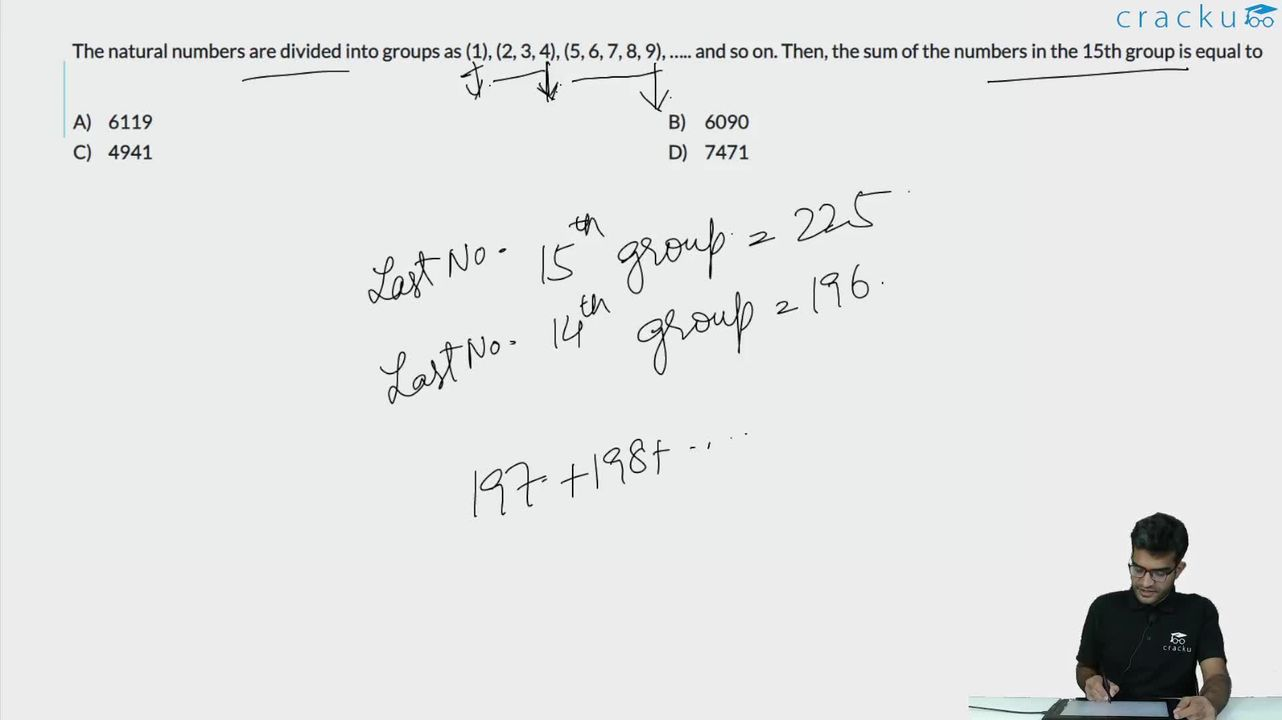Question 21

# The natural numbers are divided into groups as (1), (2, 3, 4), (5, 6, 7, 8, 9), ….. and so on. Then, the sum of the numbers in the 15th group is equal to

Solution

The first number in each group: 1, 2, 5, 10, 17.....

Their common difference is in Arithmetic Progression. Hence, the general term of the series can be expressed as a quadratic equation.

Let the quadratic equation of the general term be $$ax^2+bx+c$$

1st term = a+b+c=1

2nd term = 4a+2b+c=2

3rd term = 9a+3b+c=5

Solving the equations, we get a=1, b=-2, c=2.

Hence, the first term in the 15th group will be $$\left(15\right)^2-2\left(15\right)+2=197$$

We can see that the number of terms in each group is 1, 3, 5, 7.... and so on. These are of the form 2n-1. Hence, the number of terms in 15th group will be 29. Hence, the last term in the 15th group will be 197+29-1 = 225.

Sum of terms in group 15= $$\frac{29}{2}\left(197+225\right)\ =\ 6119$$

Alternatively,

The final term in each group is the square of the group number.

In the first group 1, second group 4, ............

The final element of the 14th group is $$\left(14\right)^2=\ 196$$, similarly for the 15th group this is : $$\left(15\right)^2=\ 225$$

Each group contains all the consecutive elements in this range.

Hence the 15th group the elements are:

(197, 198, ................................225).

This is an Arithmetic Progression with a common difference of 1 and the number of element 29.

Hence the sum is given by :  $$\frac{n}{2}\cdot\left(first\ term\ +last\ term\right)$$

$$\frac{29}{2}\cdot\left(197+225\right)$$

6119.

### View Video Solution